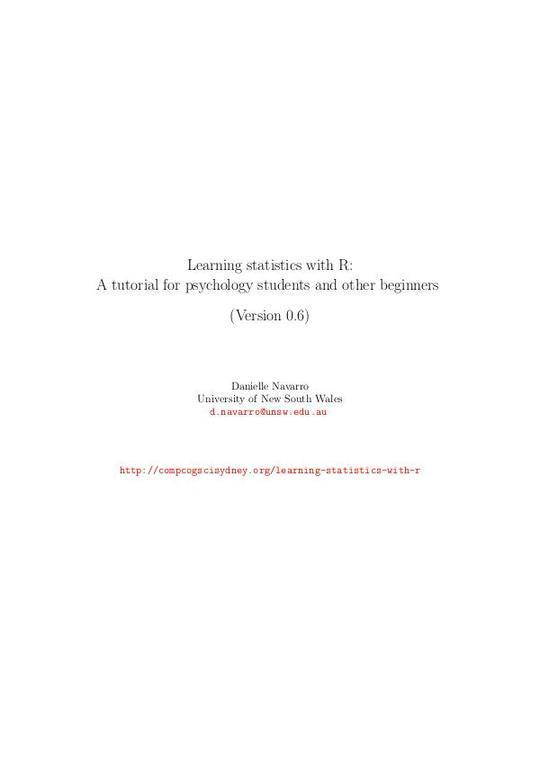Free

# Learning Statistics with R

By Daniel Navarro
Free
The publisher has enabled DRM protection, which means that you need to use the BookFusion iOS, Android or Web app to read this eBook. This eBook cannot be used outside of the BookFusion platform.
Book Description
• Preface
• I Background
• Why do we learn statistics?
• On the psychology of statistics
• The cautionary tale of Simpson's paradox
• Statistics in psychology
• Statistics in everyday life
• There's more to research methods than statistics
• A brief introduction to research design
• Introduction to psychological measurement
• Scales of measurement
• Assessing the reliability of a measurement
• The ``role'' of variables: predictors and outcomes
• Experimental and non-experimental research
• Assessing the validity of a study
• Confounds, artifacts and other threats to validity
• Summary
• II An introduction to R
• Getting started with R
• Installing R
• Typing commands at the R console
• Doing simple calculations with R
• Storing a number as a variable
• Using functions to do calculations
• Storing many numbers as a vector
• Storing text data
• Storing ``true or false'' data
• Indexing vectors
• Quitting R
• Summary
• Managing the workspace
• Navigating the file system
• Useful things to know about variables
• Factors
• Data frames
• Lists
• Formulas
• Generic functions
• Getting help
• Summary
• III Working with data
• Descriptive statistics
• Measures of central tendency
• Measures of variability
• Skew and kurtosis
• Getting an overall summary of a variable
• Descriptive statistics separately for each group
• Standard scores
• Correlations
• Handling missing values
• Summary
• Drawing graphs
• An overview of R graphics
• An introduction to plotting
• Histograms
• Stem and leaf plots
• Boxplots
• Scatterplots
• Bar graphs
• Saving image files using R and Rstudio
• Summary
• Pragmatic matters
• Tabulating and cross-tabulating data
• Transforming and recoding a variable
• A few more mathematical functions and operations
• Extracting a subset of a vector
• Extracting a subset of a data frame
• Sorting, flipping and merging data
• Reshaping a data frame
• Working with text
• Coercing data from one class to another
• Other useful data structures
• Miscellaneous topics
• Summary
• Basic programming
• Scripts
• Loops
• Conditional statements
• Writing functions
• Implicit loops
• Summary
• IV Statistical theory
• Introduction to probability
• How are probability and statistics different?
• What does probability mean?
• Basic probability theory
• The binomial distribution
• The normal distribution
• Other useful distributions
• Summary
• Estimating unknown quantities from a sample
• Samples, populations and sampling
• The law of large numbers
• Sampling distributions and the central limit theorem
• Estimating population parameters
• Estimating a confidence interval
• Summary
• Hypothesis testing
• A menagerie of hypotheses
• Two types of errors
• Test statistics and sampling distributions
• Making decisions
• The p value of a test
• Reporting the results of a hypothesis test
• Running the hypothesis test in practice
• Effect size, sample size and power
• Some issues to consider
• Summary
• V Statistical tools
• Categorical data analysis
• The 2 goodness-of-fit test
• The 2 test of independence (or association)
• The continuity correction
• Effect size
• Assumptions of the test(s)
• The most typical way to do chi-square tests in R
• The Fisher exact test
• The McNemar test
• What's the difference between McNemar and independence?
• Summary
• Comparing two means
• The one-sample z-test
• The one-sample t-test
• The independent samples t-test (Student test)
• The independent samples t-test (Welch test)
• The paired-samples t-test
• One sided tests
• Using the t.test() function
• Effect size
• Checking the normality of a sample
• Testing non-normal data with Wilcoxon tests
• Summary
• Comparing several means (one-way ANOVA)
• An illustrative data set
• How ANOVA works
• Running an ANOVA in R
• Effect size
• Multiple comparisons and post hoc tests
• Assumptions of one-way ANOVA
• Checking the homogeneity of variance assumption
• Removing the homogeneity of variance assumption
• Checking the normality assumption
• Removing the normality assumption
• On the relationship between ANOVA and the Student t test
• Summary
• Linear regression
• What is a linear regression model?
• Estimating a linear regression model
• Multiple linear regression
• Quantifying the fit of the regression model
• Hypothesis tests for regression models
• Testing the significance of a correlation
• Regarding regression coefficients
• Assumptions of regression
• Model checking
• Model selection
• Summary
• Factorial ANOVA
• Factorial ANOVA 1: balanced designs, no interactions
• Factorial ANOVA 2: balanced designs, interactions allowed
• Effect size, estimated means, and confidence intervals
• Assumption checking
• The F test as a model comparison
• ANOVA as a linear model
• Different ways to specify contrasts
• Post hoc tests
• The method of planned comparisons
• Factorial ANOVA 3: unbalanced designs
• Summary
• VI Endings, alternatives and prospects
• Bayesian statistics
• Probabilistic reasoning by rational agents
• Bayesian hypothesis tests
• Why be a Bayesian?
• Bayesian analysis of contingency tables
• Bayesian t-tests
• Bayesian regression
• Bayesian ANOVA
• Summary
• Epilogue
• The undiscovered statistics
• Learning the basics, and learning them in R
• References
You May Also Like